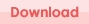# Paging Class using PHP and MySQL

Based on my last post on paging using PHP and MySQL, I have coded this class. It is very easy to implement and it will save your time. Here is a code snippet to show how simple to use it.

```//include the class file include('Pager.php');   //making connection to the database mysql_connect('localhost', 'root', ''); mysql_select_db('test');   //prepare SQL \$sql = 'SELECT * FROM books';   //create an object of Pager passing the SQL \$pager = new Pager(\$sql);   //set the url. this is the current page \$pager->url = 'index.php';   //set number of rows. by default it is 10 \$pager->rowPerPage = 5;   //build the pager \$pager->build();   //get paged data \$rows = \$pager->getPagedData();```

Click the download link given below for a copy of the class. It also includes an working example.# Paging using PHP and MySQL

I have coded a class for paging, you may find it here.

```### Code for Paging Starts ### //index no of the page \$pageIndex = ((int)\$_REQUEST['pageIndex'] == 0) ? 1 : (int)\$_REQUEST['pageIndex'];   //number of rows \$rowPerPage = 10;   //counting total number of rows \$sql = 'SELECT COUNT(*)         FROM {your_table_name}         WHERE {IF ANY CONDITION}';   \$query = mysql_query(\$sql, \$link_identifier); \$row = mysql_fetch_row(\$query);   // total records \$totalRecords = \$row;   // number of pages \$totalPages = ceil(\$totalRecords/\$rowPerPage);   if(\$pageIndex > \$totalPages) {     \$pageIndex = \$totalPages; }   \$startIndex = (\$pageIndex - 1) * \$rowPerPage;   if(\$totalRecords > \$rowPerPage) {     \$endIndex = \$startIndex + \$rowPerPage; } else {     \$endIndex = \$totalRecords; }   if(\$pageIndex == \$totalPages) {     if(\$totalRecords > \$totalPages * \$rowPerPage)     {         \$endIndex = \$totalRecords;     } }   if(\$totalRecords > 0) {     \$rangeStart = \$startIndex+1;       if((\$totalRecords > \$rowPerPage) || (\$pageIndex == \$totalPages))     {         \$rangeEnd = \$totalRecords;     }     else     {         \$rangeEnd = \$endIndex;     }       \$range = 'Showing '  . \$rangeStart.' - '.\$rangeEnd.' of '.\$totalRecords; }   // link for next page \$nextLink = 'page_name.php?pageIndex='.(\$pageIndex + 1);   // link for previous page \$previousLink = 'page_name.php?pageIndex='.(\$pageIndex - 1);   // fetching data using pagination \$sql = "SELECT *             FROM {your_table_name}             WHERE {IF ANY CONDITION}             LIMIT \$startIndex, \$rowPerPage";   \$query = mysql_query(\$sql, \$link_identifier);   ### Code for Paging Ends ###```

Now use the resource ‘\$query’ and do what you want to do.

# Enabling cURL in XAMPP

By default cURL is disable in XAMPP installation. If you want to enable cURL of your XAMPP environment then uncomment the following line:

;extension=php_curl.dll

to

extension=php_curl.dll

in the C:\Program Files\xampp\apache\bin\php.ini file (assuming you have installed XAMPP in “C:\Program Files” folder).

If you plan to switch version of PHP in XAMPP you may made the change in following files (assuming you have installed XAMPP in “C:\Program Files” folder):

• C:\Program Files\xampp\php\php5.ini
• C:\Program Files\xampp\php\php.ini
• C:\Program Files\xampp\php\php4\php.ini
• C:\Program Files\xampp\php\php4\php4.ini